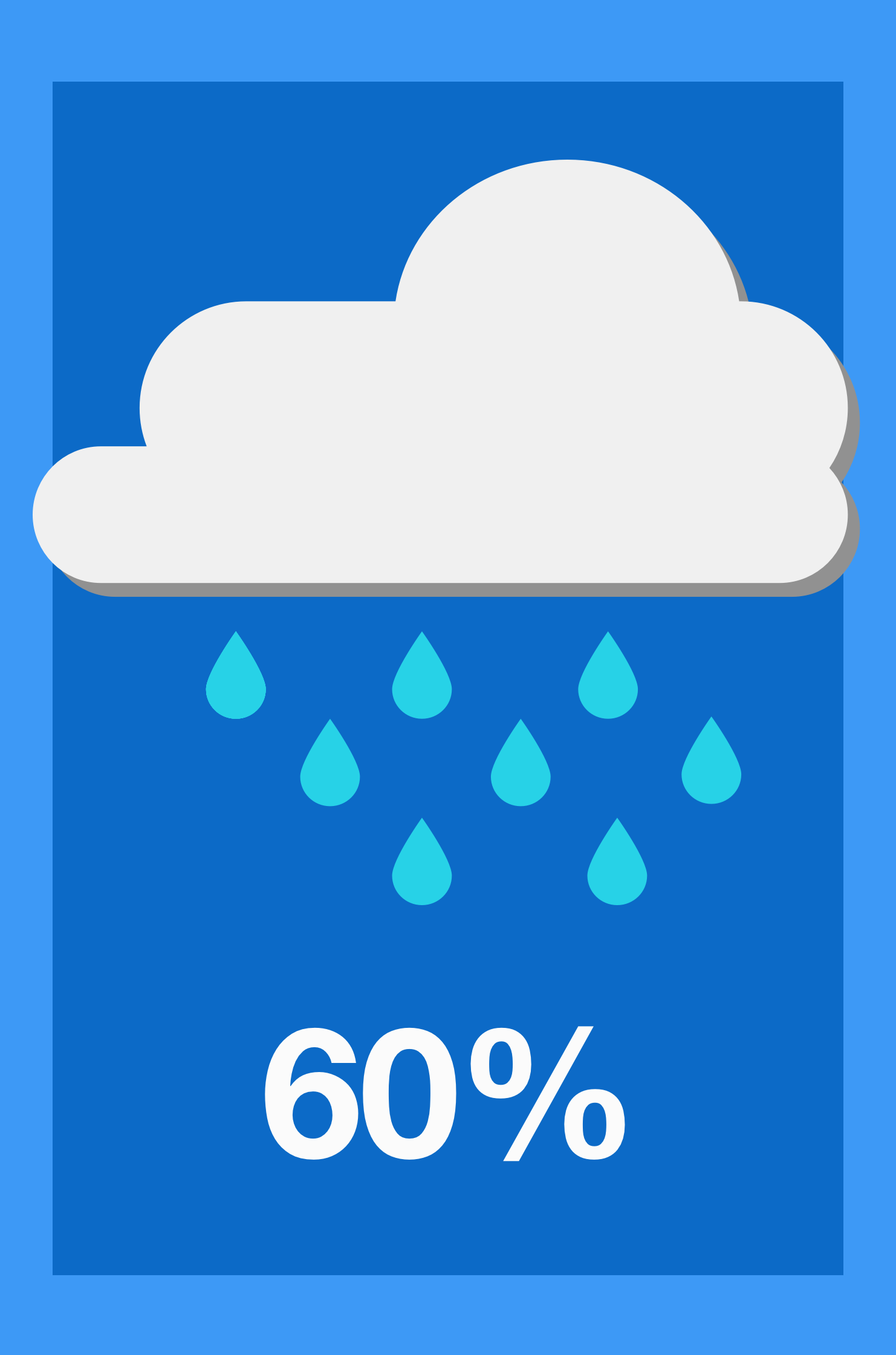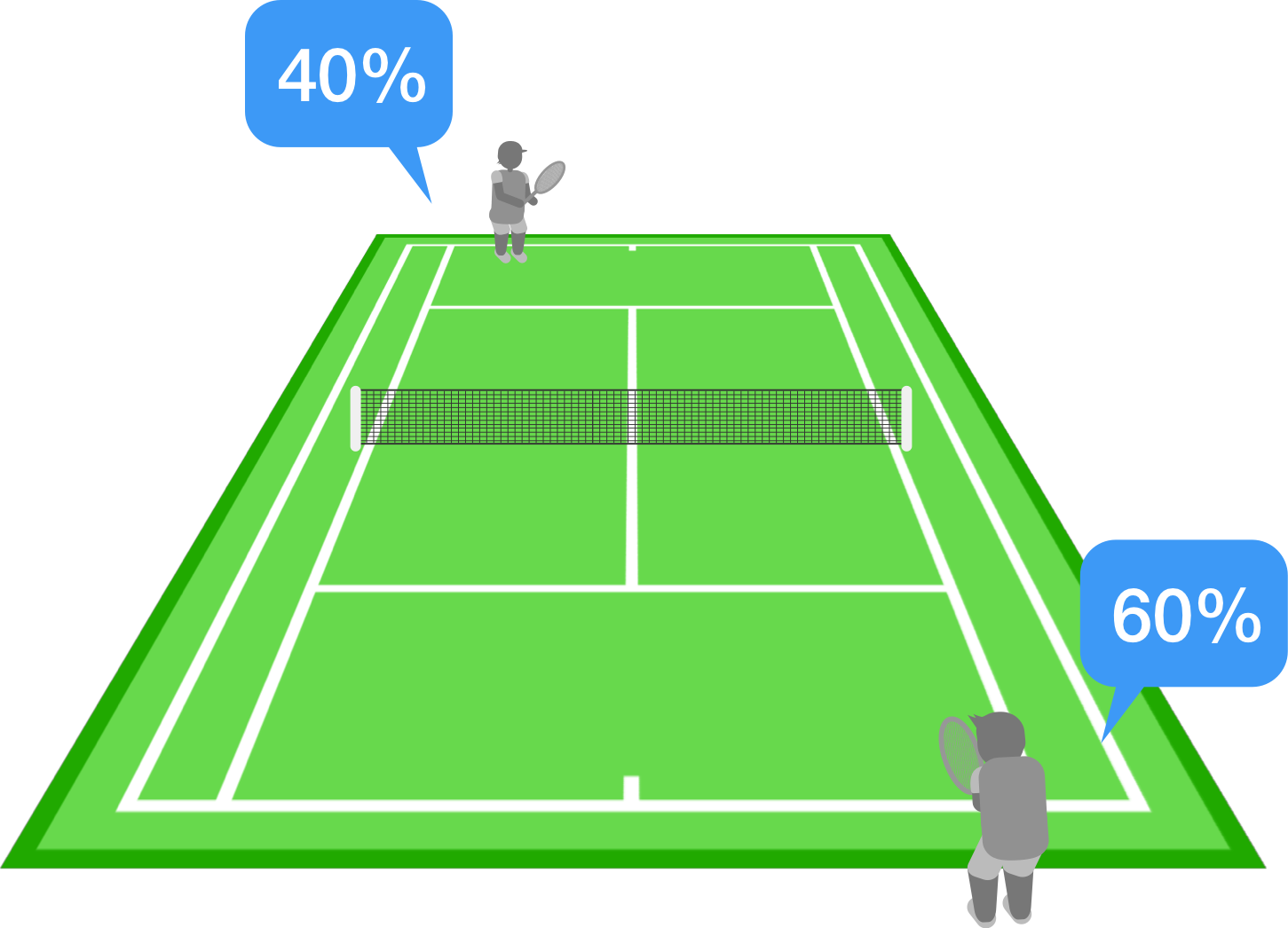### Applied Probability

We've covered a lot of ground, so it's worth taking some time to review. We'll look here at a few applications of probability drawn from physics, meteorology, and even tennis!

The problems in this quiz put the concepts of conditional probabilities, equally likely events, and counting outcomes to good use. As our intro to probability winds down, we'll also get a sense of what things are coming up in the future.

# Applications

Most scientists reject the status quo if a test result deviates from the mean by more than $2$ standard deviations. In other words, if a result is at least two standard deviations away from the mean, it is deemed as significantly far from the mean. The result can be rejected on the basis that it is a "bad" sample. The probability of a good sample significantly far from its mean (at least 2 standard deviations away) is $0.05.$

Physicists working with particles at the Large Hadron Collider, however, have stricter requirements. They only reject the status quo if a test result deviates from the mean by more than $5$ standard deviations. If the status quo is actually correct, the probability of this occurrence by chance in the normal distribution is approximately $6\times 10^{-7}$.

Physicists at the Large Hadron Collider are how many times less likely to incorrectly reject the status quo compared to most scientists?

# ApplicationsA weather forecast model predicts a 60% chance of rain in a specific area for a certain day. It does not rain in that area during that day. Which of these conclusions about the forecast model is the most reasonable?

A: With a 60% chance of rain, it should have at least rained a little bit.

B: The weather forecast model was flawed, and needs to be corrected for the future.

C: It cannot be concluded from a single day of data whether the forecast model is accurate or not.

# Applications

In WWII, enemies would engage in plane-to-plane aerial combat. Unsurprisingly, many planes were lost to crashes; if a bullet strikes a plane in a sensitive area, it's very hard to make it back to base. For the planes that did come back, the mechanics kept track of the location of bullet holes in the fuselage, so that they could reinforce the planes in the most vulnerable locations.

For American planes, the bullet holes on returning planes were distributed as follows:(Assume all parts are shot at with roughly equal frequency.) Where should the mechanics reinforce planes so that more of them come back safely?

# ApplicationsIn a certain game of tennis, Alex has a 60% probability to win any given point against Blake. The player who gets to 4 points first wins the game, and points cannot end in a tie.

What is Alex's probability to win the game? Try to use your intuition, rather than making a calculation.

# Applications

Which of the following statements is the best comparison between the probabilities used in weather forecasting and the probabilities used to describe dice rolls and coin flips?

A: Weather probabilities are completely subjective measures of likelihood, while the probabilities of dice rolls and coin flips are completely objective measurements of likelihood.

B: Weather probabilities, like the probabilities of dice rolls and coin flips, are based on objective methods and measurements. However, the probabilities of dice rolls and coin flips can be tested over many trials of the same controlled experiment, while weather probabilities cannot be tested in the same way.

C: The outcome of future weather behaves just like the outcome of the roll of a die. There are a set of weather outcomes that are each equally likely, and one of those outcomes is chosen at random.

# Applications

Real life is random, and we often have to make decisions and judgments based on limited information. Probability is one of the best mathematical tools available for navigating our uncertain world.

We touched on some of the fundamental ideas, but we need to put probability on firm ground. In the next series of quizzes, we'll learn all the basic rules at the heart of probabilistic calculations.

×